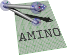amino  1.0-beta2 Lightweight Robot Utility Library
amino Namespace Reference

amino namespace More...

## Classes

struct  AxisAngle
An axis-angle object. More...

struct  DualQuat
A dual quaternion object. More...

struct  Quat
A quaternion object. More...

struct  QuatTran
A rotation quaternion and translation vector object. More...

class  RegionAllocator
An STL allocator that allocates out of a memory region. More...

struct  RegionList
Typedefs for STL lists using region allocator. More...

struct  RegionMap
Typedefs for STL map using region allocator. More...

class  RegionScope

struct  RegionVector
Typedefs for STL vector using region allocator. More...

struct  RotMat
A rotation matrix object. More...

class  ScopedMutex
Locks mutex on construction, unlocks on destruction. More...

struct  TfMat
A transformation matrix object. More...

struct  Vec3
A vector of length 3. More...

struct  XAngle
A rotation about the X axis. More...

struct  YAngle
A rotation about the Y axis. More...

struct  ZAngle
A rotation about the Z axis. More...

## Functions

template<typename T >
next (T p)

template<typename T >
prev (T p)

static struct aa_tf_vec3 operator+ (const struct aa_tf_vec3 &a, const struct aa_tf_vec3 &b)

static struct aa_tf_vec3 operator/ (const struct aa_tf_vec3 &a, double b)
Divide a Vec3 by a scalar.

static struct aa_tf_rotmat operator* (const struct aa_tf_rotmat &a, const struct aa_tf_rotmat &b)
Multiply two rotation matrices.

static struct aa_tf_quat operator* (const struct aa_tf_quat &a, const struct aa_tf_quat &b)
Multiply two ordinary quaternions.

static struct aa_tf_tfmat operator* (const struct aa_tf_tfmat &a, const struct aa_tf_tfmat &b)
Multiply two transformation matrices.

static struct aa_tf_duqu operator* (const struct aa_tf_duqu &a, const struct aa_tf_duqu &b)
Multiply two dual quaternions.

static struct aa_tf_qv operator* (const struct aa_tf_qv &a, const struct aa_tf_qv &b)
Chain two poses stored in quaternion-vector form. More...

amino namespace

## ◆ operator*()

 static struct aa_tf_qv amino::operator* ( const struct aa_tf_qv & a, const struct aa_tf_qv & b )
static

Chain two poses stored in quaternion-vector form.

It is a slight abuse of notation to use the $$*$$ symbol for this chaining as chaining quaternion-vector poses is not linear.

Definition at line 1064 of file tf.hpp.